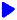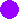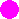Noetic Learning Math Worksheet Creator

Worksheets for PercentsBack to Worksheet Creator HomeConvert between percents and decimals    free sample

Type of conversion

Percents are

Number of questions:   questions per row:  Generate!Convert between percents and fractions       free sample

Type of conversion

Percents are

Number of questions: questions per row:  Generate!Percentage Word Problems        free sample

Type of problems

Number of questions: questions per row:  Generate!